Posted on

## New Functionality in Statistical Simulators – CC Alg II – by Kirk

Recently my good friend Joey Shavelle of Jolo Studios put some additional functionality into two of our statistical simulators. For the sample mean simulator he added code that calculates the mean of the means and the standard deviation of the means. Here’s what it looks like when I had a population mean of 127, a standard deviation of 32, and a sample size of 50 with 1000 simulations: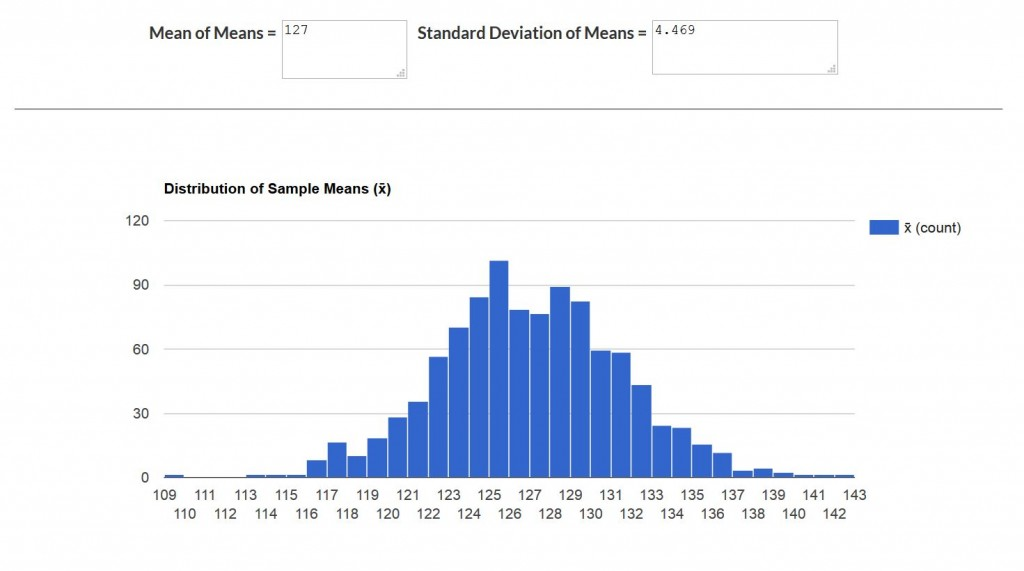(Click on the image to see it more clearly.)

He also added the same code to the sample proportion simulator that calculated the mean sample proportion and the standard deviation of the sample proportions. Here’s a shot of what it looked like when the population p was 0.3, a sample of size 100 was used, with 1000 simulations: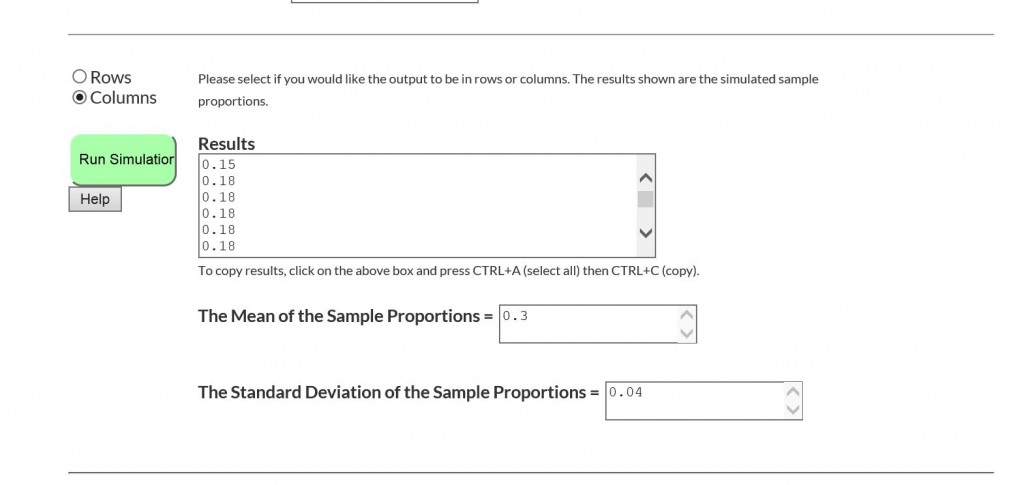So, check them out and use this new functionality in our lessons that require it if you haven’t already finished statistics at this point.

## Margin of Error

This content is only available to members.

## The Distribution of Sample Proportions

This content is only available to members.

## The Distribution of Sample Means

This content is only available to members.
Posted on

## New CC Algebra II Statistics Videos – by Kirk

A few weeks back, I published three new lessons to go with our final unit in Common Core Algebra II – Statistics. These lessons extended the work that we began in inferential statistics by introducing more formal ways of finding variation within sample statistics, such as sample means and proportions. By continuing to use simulation, we introduce students to the Central Limit Theorem for both sample means and sample proportions and extend this to a final lesson on margin of error. All of the lessons and their answer keys can be found at this post:

Simulation and Inferential Statistics – by Kirk

It took awhile, but I finally found time this weekend to record the videos for these lessons. I hope the videos are in time to help both students and teachers with this challenging material. I believe that these lessons will help students understand inferential statistics and how the results of the simulations can be extended to standard methods within statistics. Here are the YouTube links to the three new videos:

CC Alg II.Unit #13.Lesson #8.The Distribution of Sample Means

CC Alg II.Unit #13.Lesson #9.The Distribution of Sample Proportions

CC Alg II.Unit #13.Lesson #10.Margin of Error

I know that the numbering system here is in conflict with our final two lessons on regression. We will be re-numbering those last two lessons on regression as Lessons #11 and #12 respectively (as well as figuring out where they really make sense in the overall text).

Posted on

## Google Sheets for CC Alg II Statistical Simulation – by Kirk

As many of you know eMathInstruction offers online apps for performing the statistical simulations required in Common Core Algebra II. You can find links to those simulators at this post of mine:

Statistical Simulations and Inferential Statistics – by Kirk

The simulators work like a charm and I explain how to use them as well as how to use our TI based calculator programs in this YouTube video:

YouTube – Statistical Simulation Programs from eMathInstruction

One of the things I mention in the video is using Google Sheets to analyze the results of the simulations. Google Sheets is super convenient to use given that everyone has access to it. I also find it is much, much easier to use than Excel in terms of creating distribution histograms of the simulation results. Here’s a distribution of 1000 sample means that I created just now:I show how to create these in the video. But, now I’ve created three Google Sheets for the simulators that people can just copy and paste their data into. I’ll give a links in a second. One of the nice things is that it will report the mean of the results, the standard deviation of the results, the 2.5% percentile, the 5% percentile, the 95% percentile, and the 97.5% percentile (hopefully you know why these are good to have). Here’s what a screen shot looks like: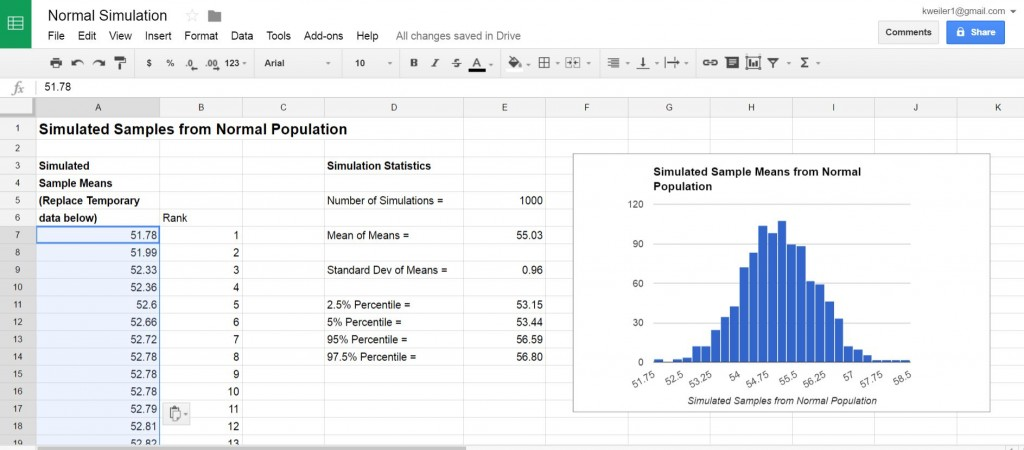As always, click on the images to see them much more clearly. Then hit the back arrow to come back to the article.

Anyhow, I’m going to supply links to all of these sheets. Now, and this is key, we don’t want to make it so that everyone is sharing and editing the same Sheet. So, I’m going to make the links “View Only.” All you have to do to have them all for yourself is make a copy: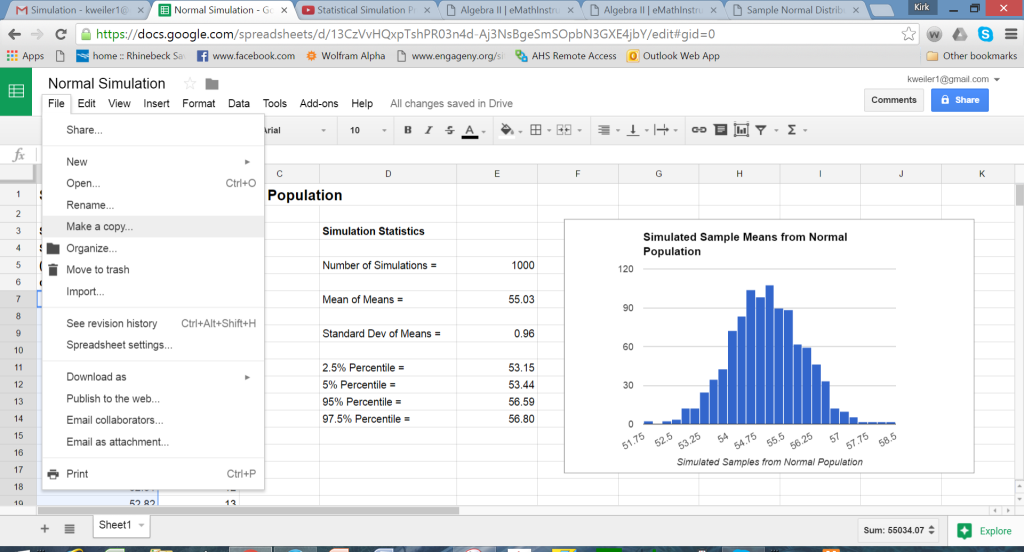Then, give it a cool new name and, as all things Google, it will magically now be in your Google Drive (assuming you have a Google account).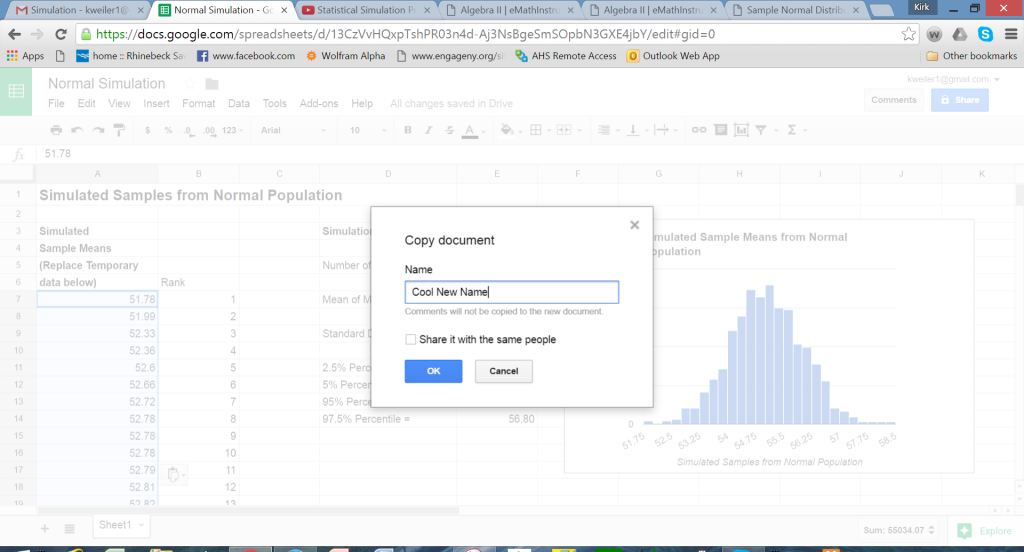Difference in Sample Means (MEANCOMP) Google Sheet

Have fun with them. If you are going to share the links with students, consider making copies on your own drive and then sharing them from there.

Posted on

## Simulation and Inferential Statistics – by Kirk

So, I’ve made quite a few posts lately because we are near the time when teachers will be working through the very confusing topic of inferential statistics in Common Core Algebra II. With so little guidance from NYSED on the matter, we are left to sift through sample problems, standards, and (ugh!) the Modules. Ultimately, inferential statistics is a topic that is much, much too large to simply squish into Algebra II.

If anything, the state should almost consider renaming the course Algebra 2 with Statistics if they are honest about the content.

What’s ultimately very difficult is that the CC Standards and the GAISE report for inferential statistics emphasize (in fact insist) on using statistical simulation instead of formulas to develop things such as confidence intervals and margins of error. The theory, at least, is that the formulas are much more understandable (in future courses) if students develop a more intuitive grasp of inferential statistics by using probabilistic thinking generated through simulation.

I’m not sure I even understood what I just typed.

Still, I do get the idea of simulation. If we find that a random sample of 50 people have an average television view time per week of 18 hours per week with a standard deviation of 3.5 hours per week, it is unlikely that the population as a whole has an average view time outside of the interval 17 to 19 hours. Here are the results of running our online simulator where I assume a population mean of 18 with a standard deviation of 3.5 and a sample size of 50. Click on the image to see it more clearly: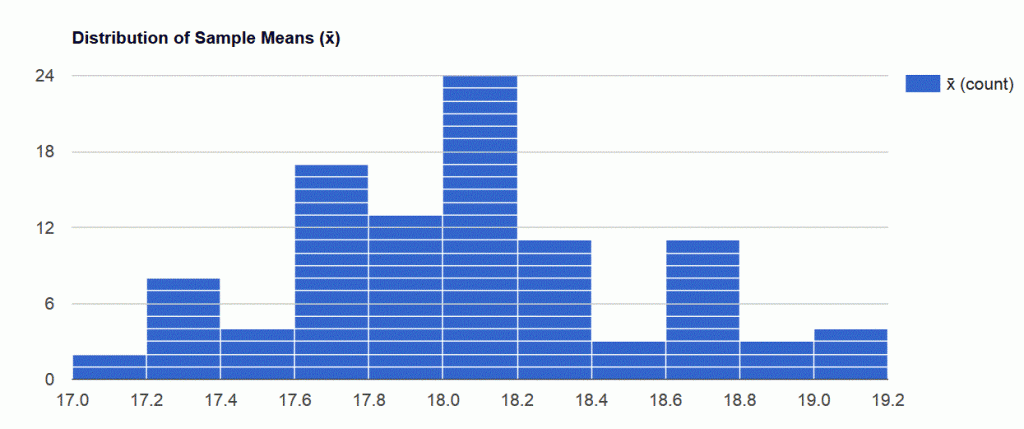Notice, there are no sample means under this simulation that fall outside of the range 17.0 to 19.2. This, in fact, would be a rough approximation for our confidence interval and half the width between these, i.e. (19.2-17.0)/2=1.1, would be a rough width for our margin of error. By the way, the actual margin of error is a theoretical 0.99 (2*stddev/sqrt(n)). Here’s a link to that simulator and our other two as well:

Sample Normal Distribution Web Based App (NORMSAMP)

Sample Proportion Simulator Web Based App (PSIMUL)

Difference of Sample Means Web Based App (MEANCOMP)

Now, there are more formulaic ways to grind out confidence intervals and margins of error. And, let’s face the fact, the state isn’t going to make them do statistical simulation on the Regents exam (June 1st); what it will do is make them interpret the results of those simulations.  I’ve created three new lessons that weren’t in Version 1 of our Common Core Algebra II text. I’ve posted them before, but I’m going to do it again, with the answer keys. Should you spend time on these more formulaic approaches to confidence interval and margin of error? That, I will leave to your professional judgement. I do tie the statistical simulation into these lessons, so that will get reinforced. Here are the lessons and their keys.

CCAlgII.Unit #13.Lesson #8.The Distribution of Sample Means

CCAlgII.Unit #13.Lesson #9.The Distribution of Sample Proportions

CCAlgII.Unit #13.Lesson #10.Margin of Error

CCAlgII.Unit #13.Lesson #8.The Distribution of Samples Means.Answer Key

CCAlgII.Unit #13.Lesson #9.The Distribution of Sample Proportions.Answer Key

CCAlgII.Unit #13.Lesson #10.Margin of Error.Answer Key

Sorry, but no videos on these yet. I am looking to do them before the end of April (so well before June 1st). Maybe consider flipping these or just giving kids the option to watch them and learn the content.

Posted on

## Statistical Simulators and Additional Lessons – Common Core Algebra II

Well, it’s getting to be crunch time in Common Core Algebra II now that April looms before us. As at Arlington, I’m sure that many districts are beginning Probability soon and will then turn to statistics in Common Core Algebra II.

I still am a little in the dark about the expectations that the state has in terms of what it wants students to know and be able to do when it comes to inferential statistics. Some of what we know comes from the CC Standards themselves, some comes from the Modules, and some comes from the sample questions. But, these three things are often contradictory.

At the end of the day, I feel like the original curriculum that I created does a good job at using Statistical Simulation to give students a good feel for how probability ties into inferential statistics. I’ve written statistical simulators for the TI-83/84 calculators and much, much faster simulators that are web-based. I describe all of these simulators in detail and supply links to the calculator code and web based apps in this earlier post of mine:

Statistical Simulation in Common Core Algebra II

I decided to add three additional lessons to the inferential statistics curriculum and am offering them up here in their pdf form. The answer keys will be available on the new answer key for CC Algebra II but aren’t available now. These three lessons take a more classic, formula based approach to inferential statistics, particularly margin of error for both means and proportions. The lessons are numbered a bit strangely because they will fit after all of the other simulation lessons but before our final two lesson on regression. Here they are:

CCAlgII.Unit #13.Lesson #8.The Distribution of Sample Means

CCAlgII.Unit #13.Lesson #9.The Distribution of Sample Proportions

CCAlgII.Unit #13.Lesson #10.Margin of Error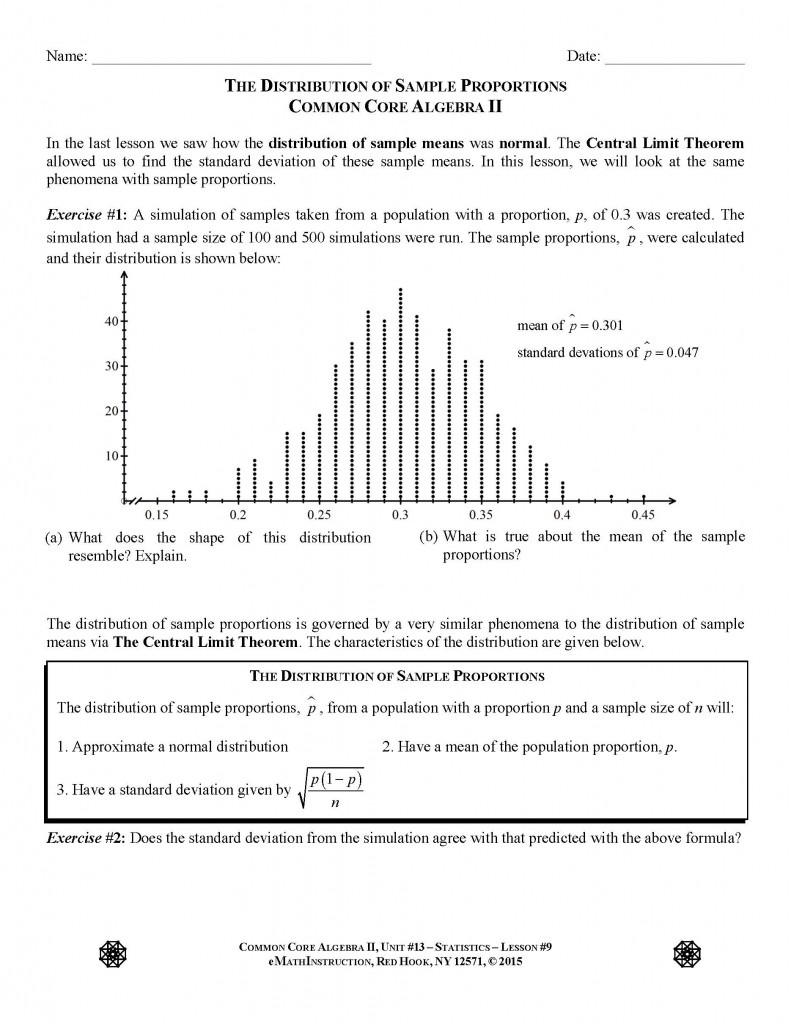## Other Types of Regression

This content is only available to members.

## Linear Regression

This content is only available to members.